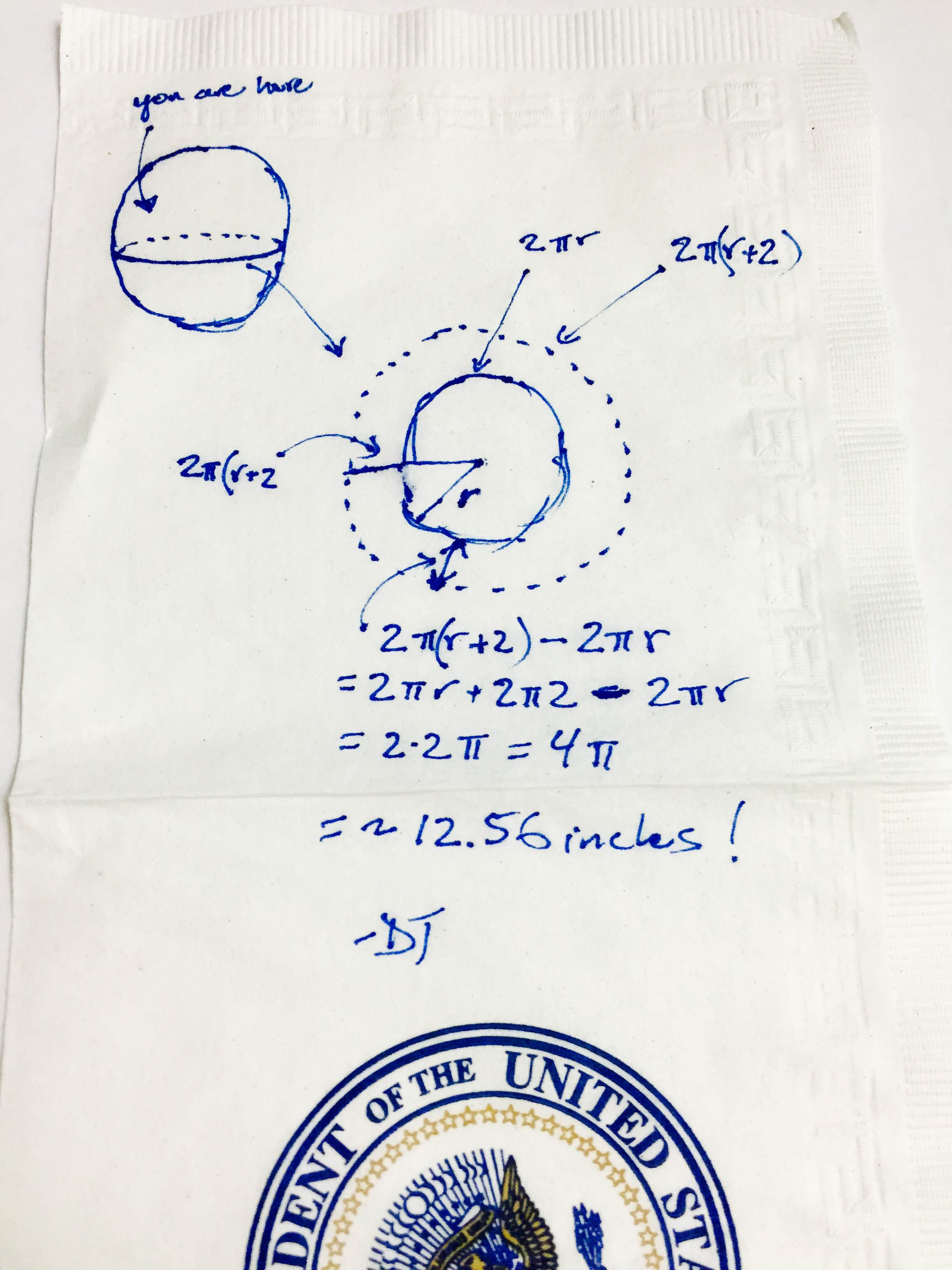This is historical material “frozen in time”. The website is no longer updated and links to external websites and some internal pages may not work.

# Back-of-the-Napkin Math, with DJ Patil: #PiDay Challenge

Summary:
U.S. Chief Data Scientist DJ Patil provides an answer to his Pi Day math challenge.On March 16, 2015, U.S. Chief Data Scientist DJ Patil scribbled out a "back-of-the-napkin" solution to a math problem he challenged the public to solve in honor of Pi Day 2015.

I hope you had an epic pi(e) day. For me, it was an opportunity to indulge in all the various forms of pi and pie (including a deconstructed apple pie). I wanted to follow up and share the answer to our pi day math brain teaser. Just a reminder, here’s the problem again:

“Imagine you have a rope snug all the way around the equator. Now you need to add some rope so that the rope is 2 inches above the ground all the way around.  How much rope do you need to add?”

The really cool thing about this problem is that it goes counter to our intuition. We think of the Earth’s equator as huge and therefore the amount of rope needed to be added must be large. But let’s do the math.

The equator of the Earth is a circle and the circumference is 2 x pi x r (or 2πr).

The radius with the height 2 inches off the ground would be r+2 inches, and the circumference would then be 2 x pi x (r+2)  (or 2π(r+2)).

Let’s subtract these to get how much rope we would need and we get 2 x pi x r - 2 x pi x (r+2).

Doing some quick math, you get 2 x pi x (r+2) - 2 x pi x r = 2 x pi x r – 2 x 2 x pi - 2 x pi x r = 4 x pi.  There you have it.

All you would have to add is 4 x pi inches or approximately 12.56 inches of rope! Crazy, but true.

That’s why you need math!

DJ Patil is U.S. Chief Data Scientist